## research papersJOURNAL OFSYNCHROTRONRADIATION
ISSN: 1600-5775
Volume 26| Part 3| May 2019| Pages 720-728

## Structure, stress and optical properties of Cr/C multilayers for the tender X-ray range

aMOE Key Laboratory of Advanced Micro-Structured Materials, Institute of Precision Optical Engineering, School of Physics Science and Engineering, Tongji University, Shanghai 200092, People's Republic of China, bDiamond Light Source Ltd, Harwell Science and Inovation Campus, Didcot OX11 0DE, UK, cSchool of Physical Science and Techology, Shanghai Tec University, 319 Middle Huaxia Road, Pudong District, Shanghai 201200, People's Republic of China, dCNR, Istituto Officina Materiali, Trieste, Italy, and eSino-German College of Applied Sciences, Tongji University, Shanghai 200092, People's Republic of China
*Correspondence e-mail: wangzs@tongji.edu.cn

(Received 19 September 2018; accepted 29 January 2019; online 1 April 2019)

Cr/C multilayer optics are a suitable choice for the tender X-ray range (1–4 keV) that covers the K absorption edges of P, S, Cl and 3d transition metals as well as the L absorption edges of 4d transition metals. In particular, these optics are studied in order to optimize the optical properties of collimated plane-grating monochromators. In this paper, the structure, stress and optical properties of Cr/C multilayers (fabricated using direct-current magnetron sputtering) with bi-layer number of 20 and the same period (about 11.64 nm) but different Cr thickness ratio (0.20–0.80) are investigated. Firstly, the grazing-incidence X-ray reflectivity at 8.04 keV was measured. These measurements were fitted assuming a multilayer structure with a four-layer and non-periodic model. Results and fitting show that interface widths increase with the Cr thickness ratio. The results obtained from X-ray diffraction at 8.04 keV were consistent with high-resolution transmission electron microscopy which showed an increase in grain size of the Cr layers. In addition, the stresses of the Cr/C multilayers have been measured and the results show that the stress value approaches zero when the Cr thickness ratio is about 0.45. The reflectivity of a Cr/C multilayer with Cr thickness ratio of 0.37 was measured and reaches 26.6% at 1.04 keV. The measured reflectivity matches very well with the predicted value using the four-layer and non-periodic model, which confirmed the viability of the prediction. Thus, the reflectivity at 1.04 keV of a Cr/C multilayer with different Cr thickness ratio was predicted and was found to drastically decrease when the Cr thickness ratio is larger than 0.37. It has been determined that a Cr thickness ratio value of 0.37 is the best choice for a Cr/C multilayer in view of high reflectivity and low stress.

### 1. Introduction

Over the last several decades, single-layer coating gratings and crystal monochromators have demonstrated excellent performance at synchrotron radiation sources in the soft and hard X-ray energy ranges (Pascarelli et al., 1996; Underwood & Gullikson, 1998; Hua et al., 2016; Martinson et al., 2017). However, in the gap range (1–4 keV), also called the tender X-ray range', where the K absorption edges of P, S, Cl and some 3d transition metals as well as the L absorption edges of 4d transition metals are located, crystal monochromators have to operate at near normal incidence because of the small d-spacing, which causes a significantly increasing power density leading to serious heat-load problems (Cowan et al., 1989). Besides, since the critical angle of total external reflection decreases drastically with photon energy (E) as 1/E, the overall efficiency of conventional single-layer coating gratings sharply reduces for photon energies above 1 keV (Jark, 2016; McNulty et al., 1997; Werner, 1977). By using multilayer-coated gratings, the overall efficiency can be increased due to the larger grazing incidence angle. Thus, multilayer-coated gratings (MLGs) were proposed to fabricate the monochromator with high efficiency in the tender range (Spiller, 1981; Rife et al., 2006; Barbee, 1989).

An important factor in the optical properties of MLGs is the substrate quality which is estimated by the precision of the groove shape and the roughness of the surface (Lin & Li, 2008). The very short wavelength in the tender X-ray spectral range requires a small blaze angle (less than 1°) and low roughness (less than 0.5 nm) which is an enormous challenge for current techniques such as diamond ruling (Hutley, 1982), holographic ion beam etching (Aoyagi & Namba, 1976), direct laser writing (Smuk & Lawandy, 1997), electron beam lithography (Wilson et al., 2003) and wet anisotropic etching (a more promising technique) (Voronov et al., 2009). Another technical challenge is achieving perfect replication of the groove profile and high-reflectivity multilayer by controlling the deposition process (Voronov et al., 2010). Here we focus on the investigation of high-reflectivity multilayers.

The efficiency of an MLG is strongly dependent on the reflectivity of the multilayer (Yang et al., 2015). For the tender X-ray range, a Cr/C multilayer can achieve a high reflectivity in principle due to the considerable difference between the refraction index of chromium and carbon and the low absorption of both materials. Therefore, the combination of a Cr/C multilayer and grating was put forward (McNulty et al., 1997). Recently, Senf et al. (2016) assembled a blazed Cr/C multilayer grating which showed a highest efficiency of 55% at 4.07 keV. Subsequently, Yang et al. (2017) designed a Cr/C multilayer-based monochromator which acquires a higher efficiency by one order of magnitude than that of the single-layer coating grating monochromator currently used at the Scanning X-ray Microscopy beamline I08 at Diamond Light Source. Simulation of a multilayer grating with a line density of 600 lines mm−1 determined by the moderate resolving power requirement shows that the efficiency reaches maximum when a blazing angle of 0.4° and multilayer period of 11.64 nm are adopted. Thus, a high-reflectivity Cr/C multilayer with a period of 11.64 nm is demanded at present.

Stress in multilayers is another critical issue for developing multilayer gratings since a large stress can lead to substrate distortion, and even coating adhesion failure (Windt, 2000). Carbon single layers deposited by different deposition techniques (cathodic arc, ion beam deposition, r.f.-plasma-enhanced chemical vapor deposition, and r.f.-sputtering) always show compressive stress (Gupta & Bhushan, 1995; Cho et al., 2011). For a Cr single layer deposited by magnetron sputtering, the stress state transforms from compression to tension with increasing argon pressure – the transition pressure is about 0.3 mtorr (Windt, 1999; Hoffman & Thornton, 1982; Windischmann, 1992). As far as we know, the stress state of Cr/C multilayers is still indistinct. However, we can deduce that the stress can be balanced near zero with a suitable choice of Cr and C layer thicknesses.

Both reflectivity and stress are strongly influenced by the multilayer structure including the interface imperfections, the state of the layers and the thickness ratio (the ratio of the thickness of an individual layer to the multilayer period). For Cr/C multilayers, the interface is relatively smooth with a roughness below 0.4 nm (Wen et al., 2015; Jiang et al., 2011). The interface widths of Cr-on-C and C-on-Cr are asymmetrical, which is explained by the energetic bombardment process and different surface free energies of Cr and C (Wen et al., 2015; Jiang et al., 2011; Borchers & Michaelsen, 2002). A Cr–C mixture exists at the interface, with a Cr:C atomic ratio of 3:2, due to the largest negative value for the enthalpy of the mixture (Tu et al., 2014; Wilson et al., 2007). However, when the base pressure is low (2 × 10−3 Pa) during the deposition process, the mixing process of Cr and C atoms is inhibited by oxides which can seriously decrease the reflectivity (Deng et al., 2009). Studies on the state of the layers show that the C layer is always in an amorphous state under the fabricating environment of different Ar pressure, and the crystallinity of the Cr layer decreases with an increase in Ar pressure (Niibe et al., 1992). The thickness ratio can significantly influence the peak intensity and full width at half-maximum (FWHM) of the reflectivity (Schuster & Gobel, 1995).

Our study was focused in particular on the fabrication of a high-reflectivity Cr/C multilayer to be used for the monochromator designed for the Diamond Light Source. Firstly, we calculated the reflectivity at 1–4 keV of the first Bragg peak of Cr/C multilayers with period 11.64 nm and different Cr thickness ratios (the ratio of the Cr layer thickness to the multilayer period). The interface was assumed to be ideally sharp. The results are shown in Fig. 1. When the Cr thickness ratio is in the 0.20–0.35 range, the reflectivity is at its highest and shows no remarkable difference. However, the reflectivity decreases drastically when the Cr thickness ratio continuously increases from 0.35 to 0.80.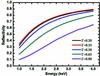Figure 1 Calculated reflectivity at 1–4 keV for C/Cr multilayers with different Cr thickness ratio Γ. The multilayer structures for the calculation have ideal interfaces. The periods and number of bilayers are 11.64 nm and 20, respectively.

The properties of a Cr/C multilayer working near the water window', normally with a period of less than 5 nm, have been studied thoroughly over the last few decades. However, a Cr/C multilayer with a much thicker period of 11.64 nm is presently demanded by the monochromator designed for the Scanning X-ray Microscopy beamline I08 at Diamond Light Source. In this paper, we present the results for Cr/C multilayers with a bi-layer number of 20, with the same period (about 11.64 nm) but with different Cr thickness ratios varying from 0.20 to 0.80. The investigation is mainly focused on the structure characterization, stress state and reflectivity of the multilayers. Finally, an optimal Cr thickness ratio is defined in view of high reflectivity and low stress.

### 2. Experiments

Five Cr/C multilayers with periods of about 11.64 nm and 20 bi-layers as well as different Cr thickness ratios (Γ = 0.23, 0.37, 0.50, 0.63, 0.80) were prepared by direct-current magnetron sputtering deposition. The samples described here were grown onto either 20 mm × 20 mm polished Si(100) wafers, which were used for structure characterizations, or, in the case of multilayers used for stress measurements, onto 0.6 mm-thick round Si(100) substrates of radius 10 mm. The base pressure was 7.0 × 10−5 Pa prior to deposition. High-purity Ar (99.999%) was used as the working gas, and during the deposition process the Ar pressure was maintained at 0.146 Pa with a closed-loop gas-flow system. The Cr layer can become oxidized in air (Odaka & Ueda, 1996; Wallwork, 1976), and a 3 nm Cr oxide layer on top can reduce the reflectivity at 1.0 keV by 7%. In order to prevent oxidation of the top Cr layer, a carbon layer of thickness 5.50 nm was deposited on top, which only reduces the reflectivity by 3%.

Grazing-incidence X-ray reflectivity (GIXRR) was measured to characterize the structure of the multilayer on a laboratory-based diffractometer (D1 system, Bede Inc.) using the Cu Kα line (λ = 0.154 nm). The GIXRR curves were first fitted with a constant-period model to characterize the actual thickness ratio. However, compared with the bandwidths and intensities of the measured Bragg peaks, the simulated Bragg peaks had narrower bandwidths and larger intensities. The same phenomenon was observed in our previous study (Wen et al., 2015). More information about the details of the multilayer structures was obtained by using a four-layer and non-periodic model which took into account the Cr–C mixture at the interface as well as the gradual changes in individual layer thicknesses of Cr and C. All fitting processes were carried out using IMD software (Windt, 1998).

Atomic force microscopy was performed on a Bruker Dimension Icon system in peak-force tapping mode over an area of 1 µm × 1 µm and the data obtained from each scan were stored in a 256 × 256 pixel array. The atomic force microscope (AFM) had a noise level of 0.002 nm, and has the ability to measure an ultra-smooth surface with high-spatial frequency roughness as low as the sub-nanometre scale. One-dimensional power spectrum density functions computed from the height data in each of the AFM images were used to figure out the differences in surface morphologies of the samples.

For the purpose of investigating the crystallization of the Cr/C multilayer, out-of-plane X-ray diffraction (XRD) measurements were carried out in asymmetrical and symmetrical reflection modes (Kobayashi, 2016). A schematic diagram is shown in Fig. 2. An X-ray beam (λ = 0.154 nm) incident on the sample surface at angle α will be diffracted into many specific directions at angle β by crystalline particles. The crystal structure can be deduced by measuring the angles 2θ (2θ = α + β) and intensities of the diffracted beams. In asymmetrical reflection mode, α was kept constant at 1°, a detector scan was carried out in the range 9–89° (i.e. the range of β was 9–89°), so that the value of 2θ was in the range 10–90°. The crystal plane detected in this mode makes an angle of 4–44° with the multilayer surface. In symmetrical reflection mode, the incident angle α varied from 5° to 45°, and the detector scanned at the angle β which was kept equal to α during the whole scanning process. The angle of 2θ was also in the range 10–90°. Only the crystal plane parallel to the multilayer surface can be detected in this mode. The crystal structure can be defined by matching the angular positions of diffraction peaks with the International Centre for Diffraction Data (ICDD) powder diffraction file.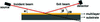Figure 2 Schematic diagram of X-ray diffraction.

The stress of the multilayer can change the curvature of the substrate. A commercial optical interferometer (λ = 632.8 nm) from Zygo Dynafiz was used to measure the radius of the substrate curvature before and after deposition. The stress can be deduced from the change of curvature radius according to Stoney's equation,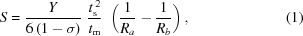where S is the stress attached to the substrate, Y is the Young's modulus of the substrate with a value of 125 GPa (Bhushan & Li, 1997), σ is Poisson's ratio of the substrate with a value of 0.279 (Kwak et al., 2003), ts is the substrate normal thickness (0.6 mm) and tm is the total thickness of the multilayer which was determined by GIXRR. Ra and Rb are the radii of substrate curvatures before and after deposition, respectively. For each Cr thickness ratio, two samples were tested and the average value was determined to represent the stress state.

The microstructure of the Cr/C multilayer with Γ = 0.37 was investigated by high-resolution transmission electron microscopy (Talos F200X) and selected-area electron diffraction (SAED). The sample for measurement was prepared using a focused ion-beam (FIB) milling system. The profile of the layers along the growth direction was obtained by reading the gray value of high-resolution transmission electron microscope (HRTEM) image using Gatan Inc.'s Digital MicrographTM software.

The reflectivity curve of the Cr/C multilayer with Γ = 0.37 was measured under a grazing angle of 3.6° on the BEAR beamline at the Elettra synchrotron source. The beamline provides linear polarized light (polarization level close to 100%) in the energy range 2.8−1600 eV.

### 3. Results

#### 3.1. Grazing-incidence X-ray reflectivity

The GIXRR measurement results of the multilayers are shown in Fig. 3by the gray dots after normalization of the reflected intensity. The angular positions of the same-order Bragg peaks are almost the same, indicating consistent periods of the multilayers. The measurement curves were fitted to infer the structure parameters. The actual thickness ratios can be obtained from fitting with the constant-period model, and the results are listed in the first column of Table 1. However, fitting with the constant-period model cannot present the details of the interfaces and thickness errors (Wen et al., 2015). According to previous studies, thickness drifts in the Cr and C layers from period to period were observed, which is caused by fluctuations in the sputtering voltage/current, argon pressure and temperature during the deposition process (Jiang et al., 2011). The Cr–C mixtures formed at the interface have a Cr:C atomic ratio of 3:2, namely of composition Cr3C2 (Tu et al., 2014; Wilson et al., 2007). Therefore, thickness drifts in the Cr and C layers were taken into account by using a non-periodic model, in which the thickness drifts were considered to be linear with the number of bi-layers. Cr3C2 interlayers at the Cr-on-C and C-on-Cr interfaces were added to the non-periodic model in consideration of the Cr–C mixtures. Thus, a four-layer and non-periodic model was adopted to perform the fitting.

 Table 1Cr thickness ratio obtained using the constant-period model, and structure parameters obtained using the four-layer and non-periodic model
Thickness (nm)
Actual Cr thickness ratio (Γ) C (capping layer) Cr top Cr bottom C top C bottom Cr-on-C (interlayer) C-on-Cr (interlayer) Period (nm) Average of interface roughness (nm)
0.23 5.65 2.01 2.03 7.78 7.84 1.20 0.72 11.75 0.32
0.37 5.65 3.36 3.40 6.12 6.16 1.34 0.80 11.66 0.34
0.50 5.57 4.60 4.65 4.53 4.55 1.41 0.97 11.54 0.36
0.63 5.44 6.09 6.13 3.11 3.19 1.50 1.08 11.84 0.40
0.80 5.46 7.62 7.64 1.11 1.14 1.66 1.20 11.61 0.49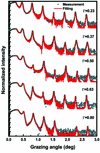Figure 3 GIXRR measurement (gray spots) and fitting curves (solid red lines) for Cr/C multilayers with a period of about 11.64 nm and bi-layer number of 20 as well as different Cr thickness ratios Γ. The fitting curves were obtained using the four-layer and non-periodic model.

The fitting curves obtained from the four-layer and non-periodic model are shown in Fig. 3by the red solid lines which match very well with the measurement curves. The structure parameter results are listed in Table 1. The carbon capping layer is about 5.50 nm thick. For Cr and C individual layers, the thicknesses of the bottom layers are slightly thicker than those of the top layers, which has also been observed in other experiments (Wen et al., 2015). We used the average values of the bottom and top layer thicknesses to represent the layer thicknesses of Cr and C. The periods were calculated by summing the layer thicknesses of Cr, C and the interlayers (Cr-on-C and C-on-Cr): the values of the periods are close to the designed value of 11.64 nm. In addition, the interfaces of the Cr/C multilayers are asymmetric, which was interpreted in previous studies (Wen et al., 2015; Jiang et al., 2011; Borchers & Michaelsen, 2002). According to Table 1, we can clearly see that the interlayer thickness increases with Cr thickness ratio (Γ), indicating that the depth of diffusion at the interface is positively related to Γ. The stronger diffusion effect can reduce the difference in refractive index between adjacent layers, causing a decrease in reflectivity. The average interface roughnesses show no evident change when Γ is less than 0.50 and significantly increase when Γ is larger than 0.50. Subsequently, we find that these variations are related to the crystallization of the Cr layers, which will be discussed in the following sections. During the fitting process the densities of Cr, C and the interlayers were set as fitting parameters because these determine the refractive indexes at a fixed photon energy (Windt, 1998). The obtained densities of Cr, C and the interlayers are close to their bulk densities, which is commonly found in sputtered films.

#### 3.2. Atomic force microscope

The surface morphologies of the Cr/C multilayers were measured by AFM. The results are shown in Figs. 4(a)–4(e). There is no obvious difference in the surface morphology of all the samples except for that with Γ = 0.80 which shows grain-like features randomly distributed over the surface. The one-dimensional power spectral density (PSD) was calculated from the AFM images, which provides a representation of the amplitude of the surface roughness as a function of spatial frequency. For the frequency fm, the PSD function along the horizontal axis (x-axis) for a digitized profile consisting of N points sampled at intervals of l is approximated by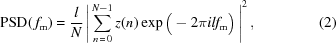where z(n) is the height of the nth point. The average of the PSDs on the vertical axis (y-axis) represents the one-dimensional PSD functions of the AFM image. Due to the isotropic surface of the multilayer, one-dimensional PSDs along the horizontal and vertical direction of the AFM images are consistent with each other. Here, we only display the PSD curves along the horizontal direction in Fig. 4(f). The surface root-mean-square (RMS) was calculated by integrating the PSD curve, and is marked in the lower-right corner of the AFM images. The PSD curves and RMS values of the samples with Γ = 0.23, 0.37 and 0.50 are nearly identical, corresponding to a consistent interface roughness. When the Cr thickness ratio increases from 0.50 to 0.63, the GIXRR fitting results show an increased interface roughness. However, the smoothing effect of the top C layer can lead to a smaller surface roughness (Peng et al., 2016). This could be the reason why the PSD curves and RMS values of the samples with Γ = 0.63 are no different from those of the samples with Γ = 0.23, 0.37 and 0.50. As for the PSD of the sample with Γ = 0.80, one prominent feature is the significant increase in the whole spatial frequency range, and the largest difference appears in the middle spatial frequency at about 13 µm−1. Thus, a sharp increase in RMS from 0.246 nm to 0.342 nm appears while the thickness ratio increases from 0.63 to 0.80. This is consistent with the GIXRR fitting results: the interface roughness increases significantly with the Cr thickness ratio increasing from 0.63 to 0.80.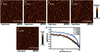Figure 4 (a)–(e) AFM images of the surface of Cr/C multilayers with different Cr thickness ratios Γ. (f) One-dimensional power spectral density (PSD) curves of the AFM images.

#### 3.3. X-ray diffraction

For investigating the crystal structure of Cr/C multilayers, out-of-plane XRD was carried out in asymmetrical and symmetrical reflection modes which were introduced specifically in the Experiment section. Fig. 5(a)shows the XRD pattern measured in asymmetrical reflection mode which was applied to determine the structure of the grains with the crystal plane not parallel to the multilayer surface. The two inserts are enlarged views of the areas marked by the black frames. The centroid angular position and the FWHM of the diffraction peaks were determined by fitting the peaks with a Gaussian. Three peaks appear for all samples except the one with Γ = 0.23 at diffraction angles of approximately 44.39°, 64.58° and 81.72°. According to the ICDD powder diffraction file, we determined that the three peaks correspond to diffractions from the (110), (200) and (211) crystal planes, respectively, in the Cr layers and the crystal structure is a body-centered cubic structure with lattice constants a = b = c = 0.2884 nm. For the sample with Γ = 0.23, both of the Cr and C layers are amorphous since there is no observable diffraction peak. As the Cr thickness ratio increases to 0.37, the diffraction peak is very wide and weak, indicating that the crystallization of the Cr layer is in the initial stage and most of the Cr layers are still close to the amorphous state. Furthermore, when the Cr thickness ratio continuously increases to 0.50, 0.63 and 0.80, the FWHM of the diffraction peak becomes narrower and the intensity is enhanced. Fig. 5(b)shows the XRD pattern measured in symmetrical reflection mode which is generally used to characterize the structure of the grains with the crystal plane parallel to the multilayer surface. Only one peak appeared for all samples except the one with Γ = 0.23 at a diffraction angle of approximately 44.39°, which is consistent with previous studies (Niibe et al., 1992). This indicates that Cr layers grow along the 〈110〉 crystal orientation. For the sample with Γ = 0.23, the XRD patterns obtained from both modes show no diffraction peaks which means that the Cr layers are amorphous. The diffraction peak intensity is proportional to the volume fraction of a phase in a mixture of phases. The intensities of the diffraction peaks from parallel-surface grains are several times larger than those from non-parallel-surface grains, which means that the volume fraction of the former grains is greater than that of the latter grains.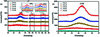Figure 5 Out-of-plane XRD results of Cr/C multilayers with different Cr thickness ratios. (a) Patterns measured in asymmetrical reflection mode. The two inserts are enlarged views of the area marked by the black frames. (b) Patterns measured in symmetrical reflection mode.

With Γ increasing, the positions of the peaks diffracted from the same crystal planes shift slightly toward the larger angles direction. The reason for the shift is that carbon atoms diffuse into the Cr layers and replace some chromium atoms in the crystal, which causes the interplanar crystal spacing to decrease (Mukhtar et al., 2012). This is evidence for the existence of the interlayer (mixture of Cr and C atoms) at the interface. The extent of the shifts increases with Cr thickness ratio, indicating that more carbon atoms diffuse into the Cr layer and replace the chromium atoms.

The size of crystal particle can be deduced from the Scherrer formula,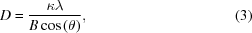where D is the mean size of the grains perpendicular to the crystal plane direction, κ is a dimensionless shape factor with a value of 0.89, λ is the X-ray wavelength equal to 0.154 nm, B is the FWHM of the diffraction peak and θ is the centroid position of the diffraction peak. Using this formula, the grain size was calculated from the patterns in Fig. 5(a). With Γ increasing from 0.37 to 0.80, the grain sizes perpendicular to the (110), (200) and (211) crystal planes increase from 3.81 nm to 8.55 nm, 8.43 nm to 11.58 nm and 8.71 nm to 12.15 nm, respectively. The larger grains cause the gaps between the grains to become wider. More space for the diffusion process is created. Thus, more carbon atoms are mixed into the Cr layer at the interface, which can increase the possibility of the process of carbon atoms replacing chromium atoms. This is why the extent of the shift and the interlayer thickness increase with increasing Cr thickness ratio. In addition, larger grains can cause the interface to be more uneven, which is responsible for the increase of interface roughness (Bagchi et al., 2009; Neuhold et al., 2011). When the Cr thickness ratio increases from 0.63 to 0.80, the intensities and FWHMs of the diffraction peaks change significantly, implying a great increase in crystallinity and grain size, which results in a substantial increase of the interface roughness.

#### 3.4. Transmission electron microscopy

Figs. 6(a) and 6(b)show cross-sectional bright-field transmission electron micrographs of the Cr/C multilayer with a Cr thickness ratio of 0.37. Bright-field imaging is a powerful tool for observing the multilayer structure, especially the interlayer region. The white stripes are C layers and the black stripes are Cr layers. Lattice fringes diffracted from Cr crystallites are easy to observe, indicating that the Cr layers are partly crystallized. The Cr and C layers are divided by the relatively thin interlayers, which can be observed more clearly in the high-resolution image [Fig. 6(b)]. The asymmetry in the Cr-on-C and C-on-Cr interlayers observed in previous studies is visible in these images. The dark-field image is displayed in Fig. 6(c). Dark-field imaging is usually used to determine the crystallites in a particular orientation. The gray layers are C and the black layers are Cr. There are several bright areas in the Cr layer, which are attributed to the diffraction of Cr grains oriented along the 〈110〉 direction. To emphasize an important point, for the polycrystalline layers, only the crystallites oriented in a certain direction can be determined in each image. Therefore, the crystallites oriented in other directions are not visible in Fig. 6(c). The SAED image obtained near the middle of the multilayer stack is shown in Fig. 6(d). Three diffraction rings appear and the radiuses of the three rings are 4.78 nm−1, 6.70 nm−1 and 8.32 nm−1, which correspond to the diffraction rings of the (110), (200) and (211) crystal planes in the Cr grains, respectively. Overall, the results of crystallites obtained from dark-field imaging and SAED are consistent with the results of XRD.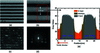Figure 6 Cross-sectional HRTEM images of a Cr/C multilayer with a Cr thickness ratio of 0.37 in the (a)–(b) bright-field and (c) dark-field. (d) The SAED pattern image. (e) The average gray value profile of an area in (b) along the growth direction of the multilayer.

Fig. 6(e)shows the average gray-value profile of the Cr/C multilayer. The y-axis represents the average gray-values along the growing direction of the multilayer. The thick interlayer causes a clear plateau at the Cr-on-C interface in the gray-value profile, and the corresponding interlayers are clearly observed in the HRTEM images. The borders between Cr, C and the interlayers are defined at the points where the slopes of the gray-value curve changes obviously. This approach gives the average interface widths of Cr-on-C and C-on-Cr of approximately 1.34 nm and 0.78 nm, respectively; and the thicknesses of the C and Cr layers are approximately 6.17 nm and 3.40 nm, respectively. These structure parameters are quantitatively similar to the fitted values of the GIXRR results, which confirms the validity of the GIXRR fitting using the four-layer and non-periodic model.

#### 3.5. Stress

Fig. 7shows the stress of a Cr/C multilayer as a function of Cr thickness ratio. The measured stress is contributed by the multilayer and capping layer, which is strongly dependent on the Cr thickness ratio. The line representing zero stress intersects the polyline at the point where the Cr thickness ratio is 0.45. We can roughly deduce that the stress approaches zero at a Cr thickness ratio of 0.45. The multilayer with a Cr thickness ratio of 0.23 exhibited a compressive stress of −530.83 MPa. As the Cr thickness ratio increases from 0.23 to 0.45, the value of the compressive stress reduces and approaches zero at a Cr thickness ratio of about 0.45. When the Cr thickness ratio increases continuously (larger than 0.45), the stress turns out to be tensile and increases to a maximum value of 1460.58 MPa at a Cr thickness ratio of 0.80. As we mentioned before, the C layer always shows compressive stress, and the Cr layer fabricated under an Ar pressure of 1.1 mtorr shows tensile stress. The mechanical stresses in the Cr and C layers compensate each other because of their opposite signs. For the structures containing thick layers of C and thin layers of Cr (small Cr thickness ratio), the stress of the C layers is significantly larger than that of the Cr layers, leading to the stress of the multilayer being compressive; whereas, with a different Cr thickness ratio close to 1, the converse situation arises. This stress transitions occurs at a Cr thickness ratio of about 0.45.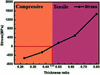Figure 7 Stress of the Cr/C multilayer versus Cr thickness ratio.

#### 3.6. Reflectivity measurement

The reflectivity curve of Cr/C multilayers with a Cr thickness ratio of 0.37 was measured under a grazing incidence angle of 3.6° on the BEAR beamline at the Elettra synchrotron radiation source. The measurement results are shown in Fig. 8(a)by the blue circles. The peak reflectivity is 26.6% at 1.04 keV. Furthermore, we used the four-layer and non-periodic model with the parameters obtained from GIXRR to calculate the reflectivity curve under a grazing angle of 3.6°. The calculated curve is shown by the red solid line in Fig. 8(a), matching the measurement curve very well. It has been demonstrated that the effect of gradual intermixing on reflectivity can be described well by the interlayer model based on consistent fitting results from GIXRR (Deng et al., 2009; Tu et al., 2014; Wen et al., 2015). Here, the validity was proved again by the consistency of the measured and calculated reflectivity of the sample with a thickness ratio of 0.37. Thus, according to the parameters listed in Table 1, we predicted the reflectivity at 1.04 keV of the Cr/C multilayers with different Cr thickness ratio, and the result is shown in Fig. 8(b). The predicted reflectivity can have slight deviations with the real one because the model is simplified, but the demonstrated variation trend of the reflectivity with increasing Cr thickness ratio should be correct. The reflectivity at a Cr thickness ratio of 0.37 is lower by 2.9% than that at a Cr thickness ratio of 0.20. The gradual decline is ascribed to the increase of the interface width. When the Cr thickness is larger than 0.37, in principle the reflectivity of the Cr/C multilayer with sharp interface decreases drastically. In the case of a rough interface, the increasing interlayer thickness and interface roughness further reduce the reflectivity. Therefore, a significant decline in reflectivity occurs when the Cr thickness ratio is larger than 0.37. The reflectivity is even lower than 5.0% at a Cr thickness of 0.80.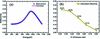Figure 8 (a) The blue circles line represents the measured reflectivity curve of the Cr/C multilayer with a thickness ratio of 0.37 under a grazing incidence angle of 3.6° at the Elettra light source. The red solid line represents the calculated reflectivity curve. (b) The calculated reflectivity at 1.04 keV of the Cr/C multilayers with different Cr thickness ratios.

### 4. Conclusion

The structure, stress and optical properties of Cr/C multilayers with different Cr thickness ratios have been investigated. The structures of the multilayers were characterized by fitting the GIXRR curves with a four-layer and non-periodic model. The results show that the interlayer thickness is positively correlated with the Cr thickness ratio; the interface roughness is almost identical for a Cr thickness ratio with a value of less than 0.50, but increases with the Cr thickness ratio when it is larger than 0.50. The variation trends are interpreted by the increasing grain size which enhances the mixing at the interface and makes the interface more uneven. TEM measurements demonstrate the structure and crystallization obtained from GIXRR and XRD. By comparing the calculated reflectivity at 1.04 keV with the measurement data, we confirmed the validity of the reflectivity prediction using the four-layer and non-periodic model. Thus, the reflectivity at 1.04 keV of the Cr/C multilayers with different Cr thickness ratios are calculated: the reflectivity decreases drastically for Cr thickness ratios larger than 0.37 because of the increasing interface widths. The Cr/C multilayer with a Cr thickness ratio of 0.37 shows compressive stress with a value of 261.72 MPa which is in the acceptable level for the monochromator. Considering the above results, a Cr thickness ratio with a value of 0.37 is the best choice for a high-reflectivity and low-stress Cr/C multilayer. The achieved Cr/C multilayer optics can promote the development of multilayer-based monochromators and high-reflectivity mirrors for the tender X-ray range. These results can assist researchers in obtaining a comprehensive knowledge of Cr/C multilayers but can also provide reference points for investigating other kinds of multilayer mirrors.

### Funding information

The following funding is acknowledged: National Key R&D Program of China (grant No. 2016YFA0401304, 2017YFA0403302); National Natural Science Foundation of China (NSFC) (grant No. 61621001, 11505129, U1732268).

### ReferencesJOURNAL OFSYNCHROTRONRADIATION